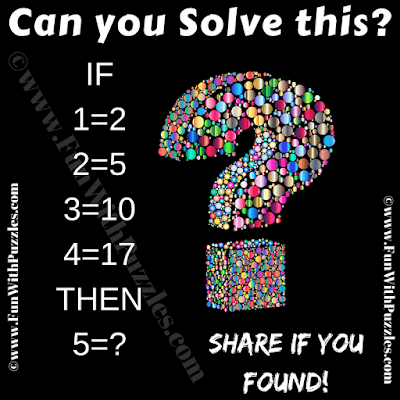## Sunday, February 17, 2019

Here is Math Logic Brain Teaser in which your challenge is find the logical pattern in the given number equations and then find the value of the missing number. Can you solve this Math Logic Puzzle without looking at the Answer?
Note that 5=2 is not correct answer. Try to decipher the hidden logic and solve this brain teaser.Can you solve this Math Logic Brain Teaser?

1.No. 5 = 2 per the definition given in the problem.

1.Even though, I tried to use different logic for this brainteaser, but your answer is perfectly fine.

2.1.